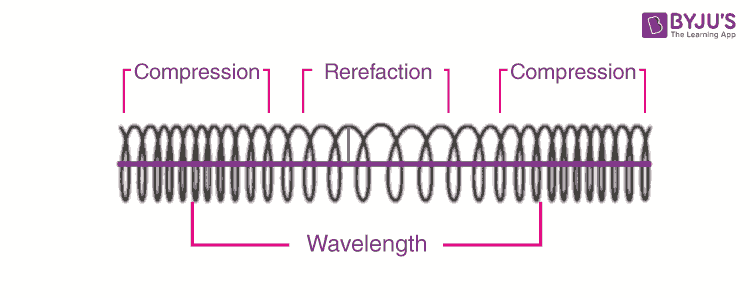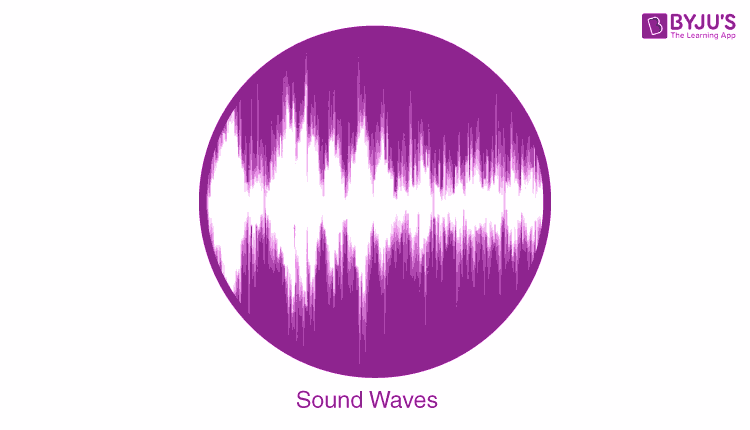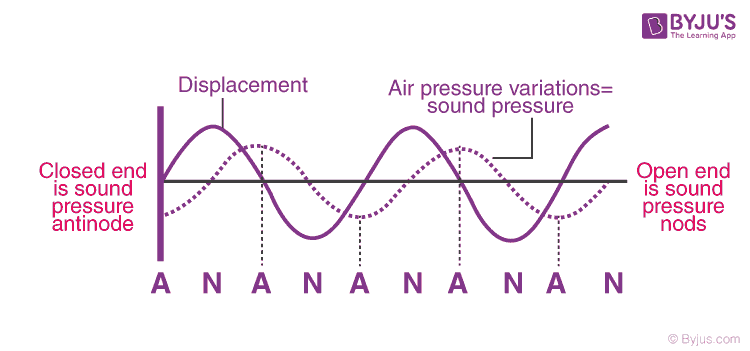# Longitudinal Waves

Mechanical waves are classified as longitudinal waves and transverse waves. Some examples of longitudinal waves are sound waves, seismic P-waves, and ultrasound waves. Transverse waves examples include electromagnetic waves and ocean waves.

 Mechanical Waves In Physics, we define wave as an oscillation about a fixed point, that is accompanied by the transfer of energy. When the transfer of energy takes place through a medium due to oscillation, the resultant wave is termed as a mechanical wave.

In this article, let us familiarize ourselves with longitudinal waves and their characteristics.

## What Is Longitudinal Wave?

Longitudinal waves are the waves where the displacement of the medium is in the same direction as the direction of the travel of the wave.

The distance between the centres of two consecutive regions of compression or the rarefaction is defined by wavelength, λ. When the compression and rarefaction regions of two waves coincide with each other, it is known as constructive interference and if the regions of compression and rarefaction do not coincide, it is known as destructive interference.## Sound Waves

A sound wave is an example of a longitudinal wave and is produced by the vibrating motion of the particles that travel through a conductive medium. An example of sound waves in a longitudinal direction is the tuning fork.

In Sound waves, the amplitude of the wave is the difference between the maximum pressure caused by the wave and the pressure of the undisturbed air. The propagation speed of sound depends upon the type, composition of the medium, and temperature through which it propagates.### Longitudinal Wave Formula

 $$y(x,t)=y_{0}cos[\omega (t-\frac{x}{c})]$$

Where,

• y is the displacement of the point on the traveling sound wave
• x is the distance the point traveled from the wave’s source
• t is the time elapsed
• yis the amplitude of the oscillations
• c is the speed of the wave
• ω is the angular frequency of the wave

Quantity x/c = time ( wave takes to travel the distance x).

Frequency (f) of the wave is given by the formula:
f=ω2π## Pressure Waves

The pressure wave is defined as the propagation of disturbance in a medium as the pressure varies.A harmonic pressure wave oscillation will be,

 y(x,t)=y0cos(kx-ωt+φ)

Where,

• y0 is the amplitude of displacement
• k is the wavenumber
• x is the distance along the axis of propagation
• ω is the angular frequency
• t is the time
• φ is the phase difference

## Characteristics of Longitudinal Waves

### Compression

In a longitudinal wave, compression is a region in which the particles of the wave are closest to each other.

### Rarefaction

Rarefaction is a longitudinal wave is when the particles are furthest apart from each other.

### Wavelength

The distance between two consecutive points in a longitudinal wave is known as wavelength. These consecutive points can be between two compressions or between two rarefactions.

### Amplitude

Amplitude is the maximum displacement of the particle from its rest point. In a longitudinal wave, the distance from the equilibrium position in the medium to compression or rarefaction is the amplitude.

### Period and Frequency

The time taken by the wave to move one wavelength is known as the period.
The frequency of the longitudinal wave is the number of wavelengths per second.

### Difference Between Longitudinal And Transverse Wave

 Longitudinal Wave Transverse Wave A wave that moves in the direction of its propagation A wave that moves in the direction perpendicular to its propagation A sound wave is an example of a longitudinal wave Water waves are an example of a transverse wave It is made of refractions and compressions It is made of troughs and crests This wave can be produced in any medium such as gas, liquid or solid This wave can be produced in solid and liquid’s surface

Stay tuned to BYJU’S and Fall in Love with Learning!

## Frequently Asked Questions – FAQs

### The longitudinal wave formula is given by the equation _____.

y(x,t)=yocos[w(t-x/c)]

### List the characteristics of sound?

The characteristics of the sound are as follows:

• Loudness
• Pitch
• Quality

### What happens when a pebble is dropped into a pond with still water?

When a pebble is dropped into a pond with still water, there is the formation of ripples on the surface of the water. These ripples are in the circular form and spread out forming alternate crests. This disturbance is caused due to the kinetic energy of the particles that oscillate and the energy gets transferred to the next layer forming ripples.

### State true or false: Mechanical waves are also known as elastic waves.

The given statement is true. Mechanical waves are also known as elastic waves because they depend on the elastic property of the waves.

### Name the condition in which sound waves can travel through the gas.

For a sound wave to travel through the gas, the required condition is an adiabatic condition. This is because when the sound waves travel they produce compressions and rarefactions which results in the generation of heat. Therefore, for the waves to travel through the gas, the temperature must be constant. Hence, the ideal condition would be an adiabatic condition.

Test your Knowledge on Logitudinal Waves